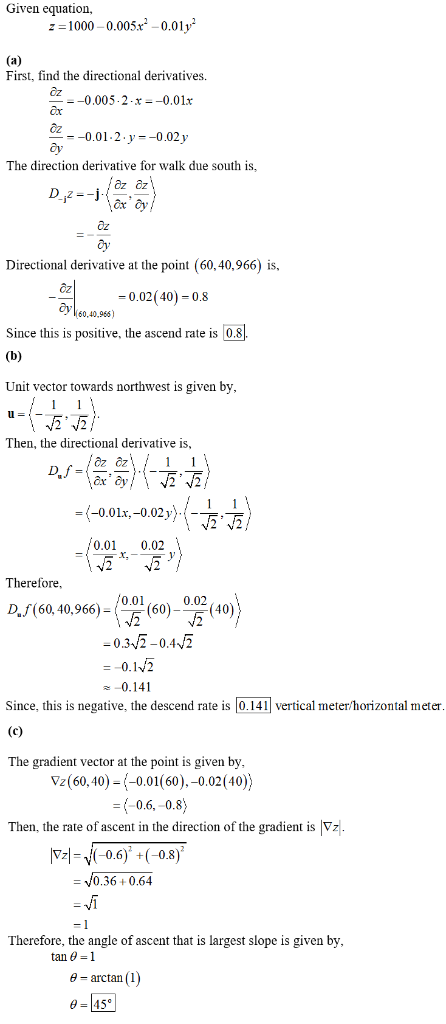# Suppose you are climbing a hill whose shape is given by the equation

Suppose you are climbing a hill whose shape is given by the
equation z=1000-0.005×2-0.01y2, where x, y, and z are measured in
meters, and you are standing at a point with coordinates
(60,40,966). The positive x-axis points east and the positive
y-axis points north. 2 2 (a) If you walk due south, will you start to ascend or
descend? At what rate? (b) If you walk northwest, will you start to ascend or
descend? At what rate? (c) In which direction is the slope largest? What is the rate
of ascent in that direction? At what angle above the horizontal
does the path in that direction begin? *Appended* I’m pretty sure that, because of part C, the whole thing has
to be treated as a function of f(x,y,z). This has no effect on the
first two answers. Also, I believe there is a mistake in parts A
and B of the first posted solution: Part A should be an ascent of
0.8m and Part B should be a descent of 0.141m. I and another person
have already arrived at those answers independently, I simply asked
the entire question so that future users will have the whole thing
on record.

Given equation, z=1000-0.005×2 -0.01v (a) First, find the directional derivatives 0.005.2 x-0.01x -0.01.2.y-0.02 y The direction derivative for walk due south is Directional derivative at the point (60,40,966) is, 0.02(40)0.8 o60.40.966) Since this is positive, the ascend rate is 0.8 (b) Unit vector towards northwest is given by, 1 Then, the directional derivative is, 1 Df åx* @y, (-0.01x,-0.02y 0.01 0,02 Therefore /0.01 0.02 (60) Daf(60, 40,966)= -0.32-0.42 =-0.12 0.14 0.141 vertical meter/horizontal meter Since, this is negative, the descend rate (c) The gradient vector at the point is given by Vz(60,40)-0.01( 60), -0.02(40)) = (-0.6, -0.8) Then, the rate of ascent in the direction of the gradient is Vz T2=-0.6) +(-0.8 = V0.36+ 0.64 = Vi 1 Therefore, the angle of ascent that is largest slope is given by, tan 1 e-arctan (1) e45°